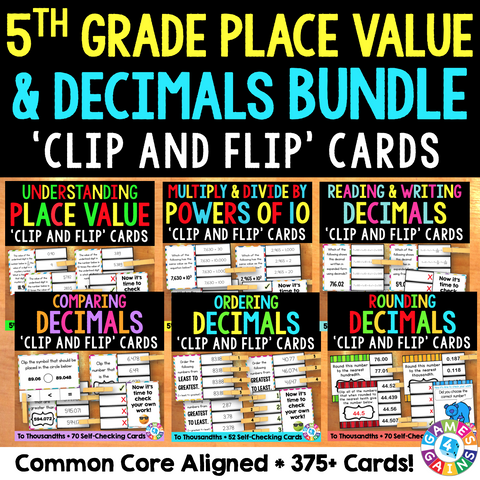## 5th Grade Place Value and Decimals 'Clip and Flip' Cards Bundle

• \$1795
• Save \$4.55

5th Grade Place Value and Decimals 'Clip and Flip' Cards Bundle contains six different sets of self-checking 'clip and flip' cards to help students practice key place value and decimal standards.

SAVE \$\$\$ when you purchase these cards with this bundle. If you bought each set individually, it would cost \$21.00!

These Place Value and Decimals 'Clip and Flip' Cards were created to support the following Common Core standards:

CCSS.MATH.CONTENT.5.NBT.A.1
Recognize that in a multi-digit number, a digit in one place represents 10 times as much as it represents in the place to its right and 1/10 of what it represents in the place to its left.

CCSS.MATH.CONTENT.5.NBT.A.2
Explain patterns in the number of zeros of the product when multiplying a number by powers of 10, and explain patterns in the placement of the decimal point when a decimal is multiplied or divided by a power of 10. Use whole-number exponents to denote powers of 10.

CCSS.MATH.CONTENT.5.NBT.A.3
Read, write, and compare decimals to thousandths.

CCSS.MATH.CONTENT.5.NBT.A.3.A
Read and write decimals to thousandths using base-ten numerals, number names, and expanded form, e.g., 347.392 = 3 × 100 + 4 × 10 + 7 × 1 + 3 × (1/10) + 9 × (1/100) + 2 × (1/1000).

CCSS.MATH.CONTENT.5.NBT.A.3.B
Compare two decimals to thousandths based on meanings of the digits in each place, using >, =, and < symbols to record the results of comparisons.

CCSS.MATH.CONTENT.5.NBT.A.4
Use place value understanding to round decimals to any place.

Want to learn more about what you'll get? Click on the links below to view the individual previews for each activity. Remember, you'll get all 6 sets when you purchase the bundle!

Understanding Place Value Clip and Flip Cards (5.NBT.1)
Contains 48 self-checking cards to help students practice identifying the relationships between adjacent digits.

Multiplying & Dividing by Powers of 10 with Exponents Clip and Flip Cards (5.NBT.2)
Contains 72 self-checking cards to help students practice multiplying and dividing whole numbers and decimals by powers of 10 (written in exponential form).

Reading and Writing Decimals Clip and Flip Cards (5.NBT.3)
Contains 64 self-checking cards to help students practice reading and writing decimals in standard form, fraction form, word form, and expanded form using decimals and fractions.

Comparing Decimals 'Clip and Flip' Cards (5.NBT.3)
Contains 70 self-checking cards to help students practice comparing decimals to tenths, hundredths, and thousandths.

Ordering Decimals to Thousandths 'Clip and Flip' Cards
Contains 52 self-checking cards to help students practice ordering decimals from least to greatest and greatest to least.

Rounding Decimals 'Clip and Flip' Cards (5.NBT.4)
Contains 70 self-checking cards to help students practice rounding decimals to the nearest hundred, ten, one, tenth, hundredth, and thousandth.

These 'clip and flip' cards are perfect for small groups and centers, or can even be used as informal assessment tools!